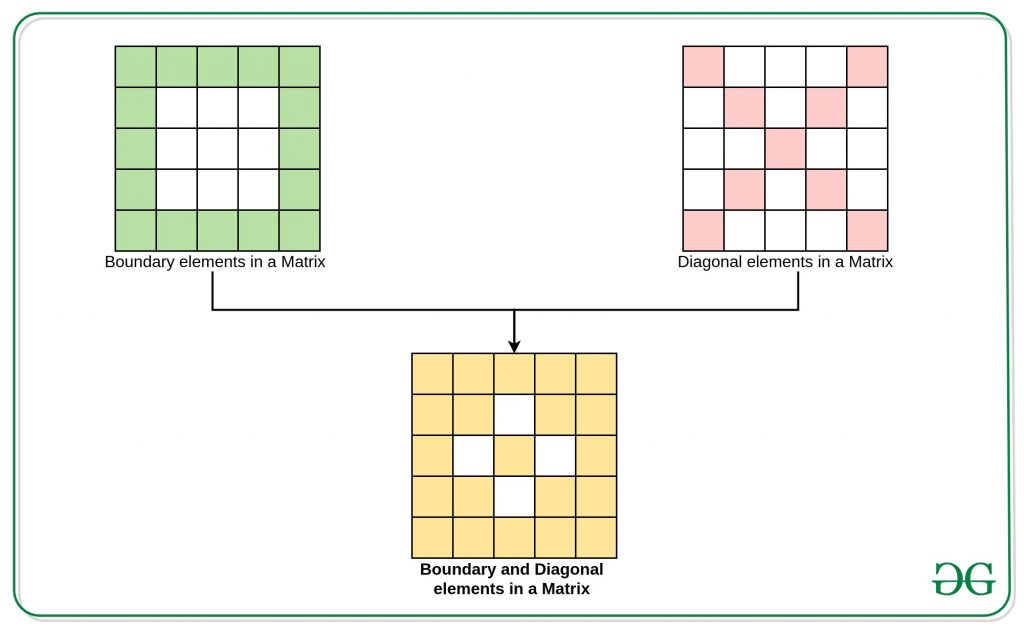GeeksforGeeks App
Open AppBrowser
Continue

# Find sum of all Boundary and Diagonal element of a Matrix

Given a 2D array arr[][] of order NxN, the task is to find the sum of all the elements present in both the diagonals and boundary elements of the given arr[][]
Examples:

Input: arr[][] = { {1, 2, 3, 4}, {1, 2, 3, 4}, {1, 2, 3, 4}, {1, 2, 3, 4} }
Output: 40
Explanation:
The Sum of elements on the boundary is 1 + 2 + 3 + 4 + 4 + 4 + 4 + 3 + 2 + 1 + 1 + 1 = 30.
The Sum of elements on the diagonals which do not intersect with the boundary elements is 2 + 3 + 2 + 3 = 10.
Therefore the required sum is 30 + 10 = 40.
Input: arr[][] = { {1, 2, 3}, {1, 2, 3}, {1, 2, 3}}
Output: 18
Explanation:
The Sum of elements on the boundary is 1 + 2 + 3 + 3 + 3 + 2 + 1 + 1 = 16.
The Sum of elements on the diagonals which do not intersect with the boundary elements is 2.
Therefore the required sum is 16 + 2 = 18.

Approach:1. Traverse the given 2D array with two loops, one for rows(say i) and another for columns(say j).
2. If i equals to j or (i + j) equals to (size of column – 1) then that element contributes to diagonals of the given 2D array.
3. If (i or j equals to 0) or (i or j equals to size of column – 1) then that element contributes to boundary elements of the given 2D array.
4. The sum of all the element satisfying above two conditions gives the required sum.

Below is the implementation of the above approach:

## C++

 `// C++ implementation of the above approach` `#include "bits/stdc++.h"``using` `namespace` `std;` `const` `int` `N = 4;` `// Function to find the sum of all diagonal``// and Boundary elements``void` `diagonalBoundarySum(``int` `arr[N][N])``{` `    ``int` `requiredSum = 0;` `    ``// Traverse arr[][]``    ``// Loop from i to N-1 for rows``    ``for` `(``int` `i = 0; i < N; i++) {` `        ``// Loop from j = N-1 for columns``        ``for` `(``int` `j = 0; j < N; j++) {` `            ``// Condition for diagonal``            ``// elements``            ``if` `(i == j || (i + j) == N - 1) {``                ``requiredSum += arr[i][j];``            ``}` `            ``// Condition for Boundary``            ``// elements``            ``else` `if` `(i == 0 || j == 0``                     ``|| i == N - 1``                     ``|| j == N - 1) {``                ``requiredSum += arr[i][j];``            ``}``        ``}``    ``}` `    ``// Print the final Sum``    ``cout << requiredSum << endl;``}` `// Driver Code``int` `main()``{``    ``int` `arr[] = { { 1, 2, 3, 4 },``                     ``{ 1, 2, 3, 4 },``                     ``{ 1, 2, 3, 4 },``                     ``{ 1, 2, 3, 4 } };` `    ``diagonalBoundarySum(arr);``    ``return` `0;``}`

## Java

 `// Java implementation of the above approach``import` `java.util.*;` `class` `GFG{``    ``public` `static` `int` `N = ``4``;``    ` `    ``// Function to find the sum of all diagonal``    ``// and Boundary elements``    ``static` `void` `diagonalBoundarySum(``int` `arr[][]){``        ``int` `requiredSum = ``0``;``        ` `        ``// Traverse arr[][]``        ``// Loop from i to N-1 for rows``        ``for` `(``int` `i = ``0``; i < N; i++) {``    ` `            ``// Loop from j = N-1 for columns``            ``for` `(``int` `j = ``0``; j < N; j++) {``    ` `                ``// Condition for diagonal``                ``// elements``                ``if` `(i == j || (i + j) == N - ``1``) {``                    ``requiredSum += arr[i][j];``                ``}``    ` `                ``// Condition for Boundary``                ``// elements``                ``else` `if` `(i == ``0` `|| j == ``0` `|| i == N - ``1``|| j == N - ``1``) {``                    ``requiredSum += arr[i][j];``                ``}``            ``}``        ``}``    ` `        ``// Print the final Sum``        ``System.out.println(requiredSum);``    ``}``    ` `    ``// Driver Code``    ``public` `static` `void` `main(String args[])``    ``{``        ``int` `arr[][] = { { ``1``, ``2``, ``3``, ``4` `},{ ``1``, ``2``, ``3``, ``4` `},``                        ``{ ``1``, ``2``, ``3``, ``4` `},{ ``1``, ``2``, ``3``, ``4` `} };``    ` `        ``diagonalBoundarySum(arr);``        ` `    ``}``}` `// This code is contributed by AbhiThakur`

## Python3

 `# Python implementation of the above approach` `N ``=` `4``;` `# Function to find the sum of all diagonal``# and Boundary elements``def` `diagonalBoundarySum(arr):``    ``requiredSum ``=` `0``;` `    ``# Traverse arr``    ``# Loop from i to N-1 for rows``    ``for` `i ``in` `range``(N):` `        ``# Loop from j = N-1 for columns``        ``for` `j ``in` `range``(N):` `            ``# Condition for diagonal``            ``# elements``            ``if` `(i ``=``=` `j ``or` `(i ``+` `j) ``=``=` `N ``-` `1``):``                ``requiredSum ``+``=` `arr[i][j];``            ` `            ``# Condition for Boundary``            ``# elements``            ``elif``(i ``=``=` `0` `or` `j ``=``=` `0` `or` `i ``=``=` `N ``-` `1` `or` `j ``=``=` `N ``-` `1``):``                ``requiredSum ``+``=` `arr[i][j];` `    ``# Print the final Sum``    ``print``(requiredSum);`  `# Driver Code``if` `__name__ ``=``=` `'__main__'``:``    ``arr ``=` `[[ ``1``, ``2``, ``3``, ``4` `],``    ``[ ``1``, ``2``, ``3``, ``4` `],``    ``[ ``1``, ``2``, ``3``, ``4` `],``    ``[ ``1``, ``2``, ``3``, ``4` `]];` `    ``diagonalBoundarySum(arr);` `# This code is contributed by 29AjayKumar`

## C#

 `// C# implementation of the above approach``using` `System;` `class` `GFG``{``    ``public` `static` `int` `N = 4;``    ` `    ``// Function to find the sum of all diagonal``    ``// and Boundary elements``    ``static` `void` `diagonalBoundarySum(``int``[, ] arr){``        ``int` `requiredSum = 0;``        ` `        ``// Traverse arr[][]``        ``// Loop from i to N-1 for rows``        ``for` `(``int` `i = 0; i < N; i++) {``    ` `            ``// Loop from j = N-1 for columns``            ``for` `(``int` `j = 0; j < N; j++) {``    ` `                ``// Condition for diagonal``                ``// elements``                ``if` `(i == j || (i + j) == N - 1) {``                    ``requiredSum += arr[i,j];``                ``}``    ` `                ``// Condition for Boundary``                ``// elements``                ``else` `if` `(i == 0 || j == 0 || i == N - 1|| j == N - 1) {``                    ``requiredSum += arr[i,j];``                ``}``            ``}``        ``}``    ` `        ``// Print the final Sum``        ``Console.WriteLine(requiredSum);``    ``}``    ` `    ``// Driver Code``    ``public` `static` `void` `Main()``    ``{``        ``int``[, ] arr = { { 1, 2, 3, 4 },{ 1, 2, 3, 4 },{ 1, 2, 3, 4 },{ 1, 2, 3, 4 } };``    ` `        ``diagonalBoundarySum(arr);``        ` `    ``}``}` `// This code is contributed by abhaysingh290895`

## Javascript

 ``

Output:

`40`

Time complexity: O(N*N) for given array of N*N size

Auxiliary space: O(1)

My Personal Notes arrow_drop_up